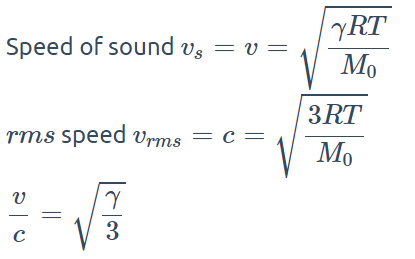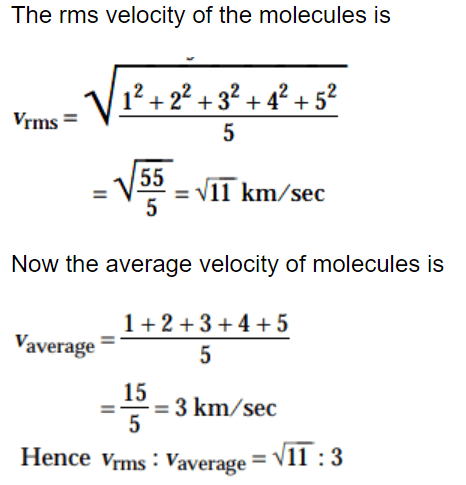## Kinetic Theory Questions and Answers Part-12

1. Speed of sound in a gas is v and r.m.s. velocity of the gas molecules is c. The ratio of v to c is
a) $\frac{3}{\gamma}$
b) $\frac{\gamma}{3}$
c) $\sqrt{\frac{3}{\gamma}}$
d) $\sqrt{\frac{\gamma}{3}}$

Explanation:2. The molecules of a given mass of a gas have a r.m.s. velocity of 200 m/sec at 27°C and $1.0\times10^{5} N\diagup m^{2}$   pressure. When the temperature is 127°C and pressure is $0.5\times10^{5} N\diagup m^{2}$  , the r.m.s. velocity in m/sec will be
a) $\frac{100\sqrt{2}}{3}$
b) $100\sqrt{2}$
c) $\frac{400}{\sqrt{3}}$
d) None of the above

Explanation:3. The average speed v and r.m.s. speed $\bar{v}$ of the molecules are related as
a) $\bar{v}=0.92v$
b) $\bar{v^{2}}=0.29v^{2}$
c) $\bar{v}=v$
d) $v=0.92\bar{v}$

Explanation: The average speed v and r.m.s. speed $\bar{v}$ of the molecules are related as $v=0.92\bar{v}$

4. The respective speeds of five molecules are 2, 1.5, 1.6, 1.6 and 1.2 km/sec. The most probable speed in km/sec will be
a) 2
b) 1.58
c) 1.6
d) 1.31

Explanation:5.At which temperature the velocity of $O_{2}$ molecules will be equal to the velocity of $N_{2}$ molecules at 0°C
a) 40°C
b) 93°C
c) 39°C
d) Cannot be calculated

Explanation:6. The respective speeds of the molecules are 1, 2, 3, 4 and 5 km/sec. The ratio of their r.m.s. velocity and the average velocity will be
a) $\sqrt{11} : 3$
b) $3 : \sqrt{11}$
c) 1 : 2
d) 3 : 4

Explanation:7. Two vessels having equal volume contains molecular hydrogen at one atmosphere and helium at two atmospheres respectively. If both samples are at the same temperature, the mean velocity of hydrogen molecules is
a) Equal to that of helium
b) Twice that of helium
c) Half that of helium
d) $\sqrt{2}$ times that of helium

Explanation: Two vessels having equal volume contains molecular hydrogen at one atmosphere and helium at two atmospheres respectively. If both samples are at the same temperature, the mean velocity of hydrogen molecules is $\sqrt{2}$ times that of helium

8. The temperature of an ideal gas is increased from 27°C to 927°C. The root mean square speed of its molecules becomes
a) Twice
b) Half
c) Four times
d) One-fourth

Explanation: The temperature of an ideal gas is increased from 27°C to 927°C. The root mean square speed of its molecules becomes twice

9. According to the kinetic theory of gases the r.m.s. velocity of gas molecules is directly proportional to
a) T
b) $\sqrt{T}$
c) $T^{2}$
d) $1\diagup \sqrt{T}$

Explanation: According to the kinetic theory of gases the r.m.s. velocity of gas molecules is directly proportional to $\sqrt{T}$